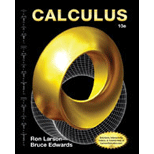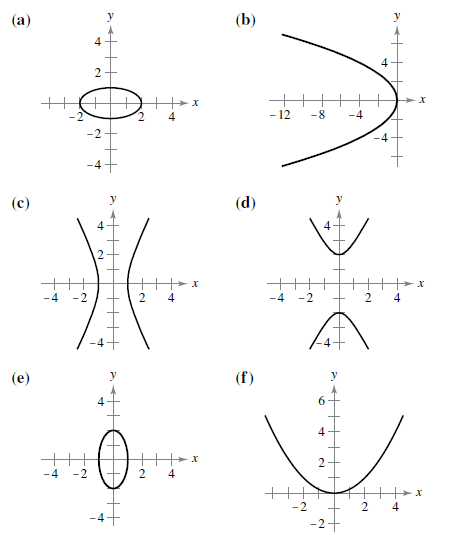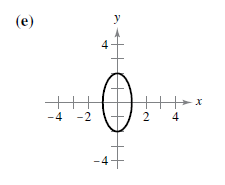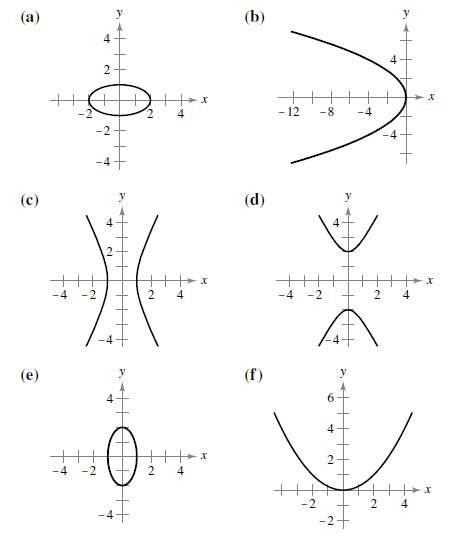# If the equation 4 x 2 + y 2 = 4 matches with its graph:### Calculus

10th Edition
Ron Larson + 1 other
Publisher: Cengage Learning
ISBN: 9781285057095### Calculus

10th Edition
Ron Larson + 1 other
Publisher: Cengage Learning
ISBN: 9781285057095

#### Solutions

Chapter 10, Problem 1RE
To determine

## If the equation 4x2+y2=4 matches with its graph:Expert Solution

Solution:

The equation 4x2+y2=4 matches with graph (e) and the graph is### Explanation of Solution

Given:

The options are:Explanation:

Here, x and y both are squared terms and both of their coefficients are positive. Thus, this equation represents an ellipse.

Now, in order to find whether the ellipse is horizontal or vertical, represent the equation in the standard form of an ellipse.

The given equation is:

4x2+y2=4

Divide the equation by 4:

x2+14y2=1

Rearrange it in standard form as:

x212+y222=1

So,

(xh)2b2+(yk)2a2=1

Which is the standard equation for an ellipse, whose major axis is vertical. That is the center of ellipse is (h,k)=(0,0) with semi axis a=1,b=2, focus is (0,3) and vertex be (0,2)

Thus, the correct graph matches for the equation that holds the above properties is option (e).

### Want to see more full solutions like this?

Subscribe now to access step-by-step solutions to millions of textbook problems written by subject matter experts!

### Want to see more full solutions like this?

Subscribe now to access step-by-step solutions to millions of textbook problems written by subject matter experts!